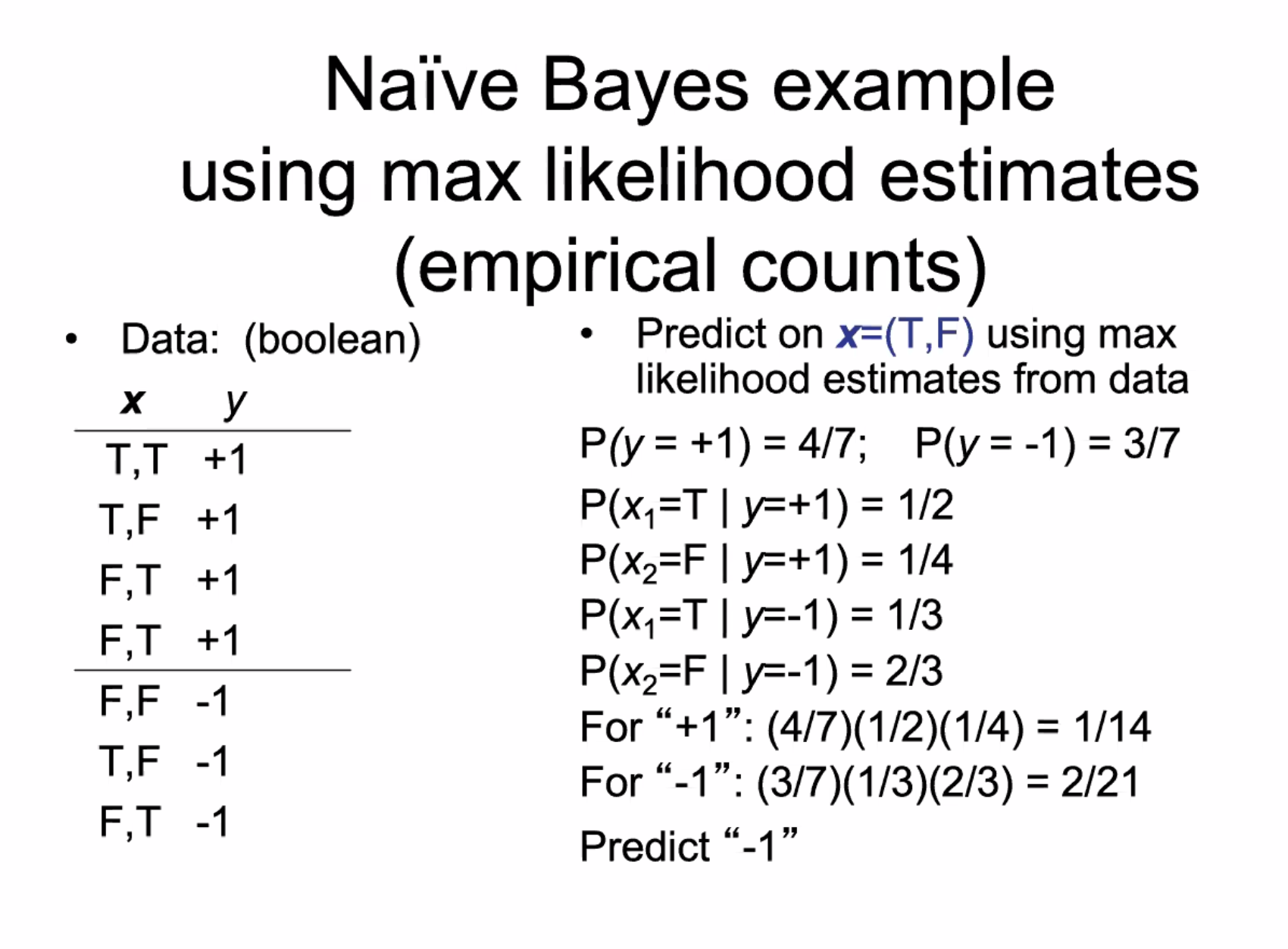# Naive Bayes¶

TLDR: predict the likelihood of the label, given features

$\begin{split}& \arg \max_y P(y | \mathbf{x}) \\ & = \arg \max_y P(\mathbf{x} | y) \frac{P(y)}{P(\mathbf{x})} \\ & = \arg \max_y P(\mathbf{x} | y) P(y)\end{split}$

Naive independence assumption: the attributes are conditionally independent given y, i.e.

$P(\mathbf{x} | y) = \prod_j P(x_j | y)$

So, we predict the label y that maximizes

$P(y) \prod_j P(x_j | y)$

This uses a generative model: pick y then generate x based on y

To implement naive bayes, we need to estimate:

• $$P(y)$$ distribution
• for each class y, for each feature $$x_j$$, need $$P(x_j | y)$$ distributions

all of these features are 1-dimensional - the combination of them is the model## Issues¶

• conditional independence is optimistic
• what if an attribute-value pair is not in the training set?
• laplace smoothing / dummy data
• continuous features: use gaussian or other density?
• attributes for text classification?
• bag of words model

## NB for Text¶

• let $$V$$ be the vocabulary (all words/symbols in training docs)
• for each class $$y$$, let $$Docs_y$$ by the concatenation of all docs labelled y
• for each word $$w$$ in $$V$$, let $$\#w(Docs_y)$$ be the number of times $$w$$ occurs in $$Docs_y$$
• set $$P(w | y) = \frac{\#w(Docs_y) + 1}{|V| + \sum_w \#w(Docs_y)}$$ (Laplacian smoothing)